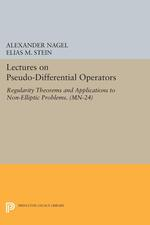Have library access? Log in through your library# Lectures on Pseudo-Differential Operators: Regularity Theorems and Applications to Non-Elliptic Problems. (MN-24)

ALEXANDER NAGEL
ΕLIAS M. STEIN
Copyright Date: 1979
Pages: 168
https://www.jstor.org/stable/j.ctt130hk7z

## Table of Contents

1. Front Matter
(pp. [i]-[ii])
2. Table of Contents
(pp. [iii]-[iv])
3. Preface
(pp. [v]-[vi])
4. INTRODUCTION
(pp. 1-6)

The object of this monograph is to present the theory of certain new classes of pseudo-differential operators. These classes of operators are intended to satisfy two general requirements. On the one hand, they should be restrictive enough to be bounded in LpLipschitz spaces, and Sobolev spaces. On the other hand, they should be large enough to allow for a description of the parametricies of some interesting non-elliptic differential and pseudo-differential operators and other operators such as the Cauchy-Szegö and Henkin-Ramirez integrals for strictly pseudo-convex domains.

Before discussing these new classes however, we will briefly recall some basic definitions, and...

5. Chapter I. Homogeneous distributions
(pp. 7-30)

Chapter I may be thought of as a review of some known facts which are basic in motivating our theory. Proofs are for the most part only sketched.

Denote x = (x1, …, xn). Fix positive exponebts a1, …, an, and define${{\delta }_{\text{t}}}(\text{x})=({{\text{t}}^{{{\text{a}}_{1}}}}{{\text{x}}_{1}},\ldots ,{{\text {t}}^{{{\text{a}}_{\text{n}}}}}{{\text{x}}_{\text{n}}}), 0 < t < \infty$. Observe that

(1) δs∘ δst

(2) δ1= Id

(3) δt(x) → 0 as t → 0 for all x

(More generally, one might let δtbe given by multiplication by the matrix eAlogtwhere A is a real matrix whose eigenvalues all have positive real part.)

The change of variable formula for dilations δt...

6. Chapter II. Basic Estimates for Pseudo Differential Operators
(pp. 31-75)

We will now consider further the above examples in order to guess the appropriate hypotheses for a relevant class of pseudo differential operators. Recall that a pseudo differential operator denoted a(x, D) is given by$\text {f}\to \int\limits_{{{\mathbb{R}}^{\text n}}}{\text {a}(\text {x},\xi ){{\text e}^{-2\Pi \text {ix}\xi }}}\hat{\text f}(\xi )\text {d}\xi$where$\hat{\text f}(\xi )=\int\limits_{{{\mathbb{R}}^{\text n}}}{{{\text e}^{2\Pi \text {ix}\xi }}}\text f(\text {x})\text {dx}$and$\text{f}\in \cal{S}$. We will assume that the symbol a(x, ξ) is smooth everywhere. (It is harmless to throw away the singularity in ξ near the origin.) Since we are only concerned with local theorems, we will also assume that a(x, ξ) has compact support in x.*classical symbols$\text {a}(\text {x},\xi )\in \text {S}_{1}^{\text m},0$satisfy

(1)$\left| \partial _{\xi }^{\alpha }\partial _{\text x}^{\beta }\text {a}(\text {x},\xi ) \right|\le {{\text C}_{\alpha ,\beta }}{{(1+\left\| \xi \right\|)}^{\text {m}-\left| \alpha \right|}}$,

where$\left\| \xi \right\|$denotes Euclidean norm. More generally,$S_{\rho ,\delta }^{m}$...

7. Chapter III. Further Regularity Theorems and Composition of Operators
(pp. 76-103)

Let$\text{L}_{\text{k}}^{\text{P}}$denote the usual Sobolev space on${{\mathbb{R}}^{\text{n}}}$, 1 < P < ∞, k real. (See e.g., Stein , Chapter 5.)

Theorem 11. If$\text{a}\in \text{S}_{\rho }^{\text{m}}$, m≤0, then a(x,D) is bounded,$\text{L}_{\text{k}}^{\text{p}}\to \text{L}_{\text{k-m/2}}^{\text{P}}$, k≥0

Lemma 1. Suppose is P(x, ξ) is a polynomial in ξ of degree < k with smooth coefficients with compact support in x. Then$\text{p}\left( \text{x},\xi \right)\text{S}_{\rho }^{2\text{k}}$.

Proof. The product of a symbol of order m1and a symbol of order m2is a symbol of order m1+ m2. A function$\text{a}\left( \text{x} \right)\in \text{C}_{0}^{\infty }$is clearly a symbol in$\`{{S}}\,_{\rho }^{0}$. Therefore, it suffices to show that${{\xi }_{\text{j}}}\in \text{S}_{\rho}^{2}$....

8. Chapter IV. Applications
(pp. 104-143)

We shall now present the main applications of our theory. Our first example arises in the setting of a domain${\cal{D}}$in ℂn+1with smooth strictly pseudo-convex boundary. We intend to show that the Cauchy-Szegö projection operator (as well as the related Henkin-Ramirez operators) are given by operators with symbols in the class$S_{\rho }^{0}$, for appropriateρ. Similarly, parametricies for${{\square }_{b}}$(acting on q-forms, where 0 < q < n) are given in terms of symbols of class$S_{\rho }^{-2}$. The proof of these facts proceeds as follows. First, natural coordinates are introduced at each point of the boundary of${\cal{D}}$...

9. Appendix: Some computations concerning the class $\text{S}_{\rho }^{\text{m}}$
(pp. 144-155)
10. References
(pp. 156-159)
11. Back Matter
(pp. 160-160)